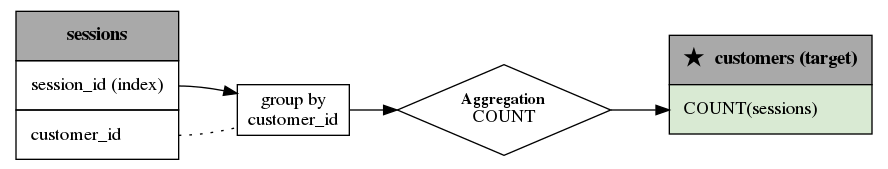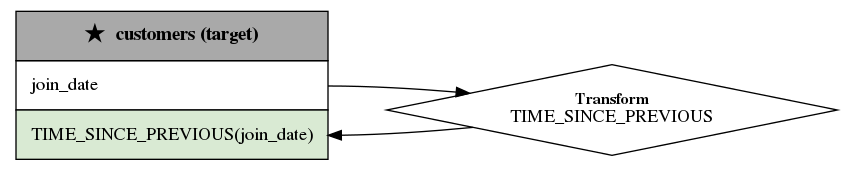NOTICE

The upcoming release of Featuretools 1.0.0 contains several breaking changes. Users are encouraged to test this version prior to release by installing from GitHub:

pip install https://github.com/alteryx/featuretools/archive/woodwork-integration.zip

For details on migrating to the new version, refer to Transitioning to Featuretools Version 1.0. Please report any issues in the Featuretools GitHub repo or by messaging in Alteryx Open Source Slack.

# Feature primitives¶

Feature primitives are the building blocks of Featuretools. They define individual computations that can be applied to raw datasets to create new features. Because a primitive only constrains the input and output data types, they can be applied across datasets and can stack to create new calculations.

## Why primitives?¶

The space of potential functions that humans use to create a feature is expansive. By breaking common feature engineering calculations down into primitive components, we are able to capture the underlying structure of the features humans create today.

A primitive only constrains the input and output data types. This means they can be used to transfer calculations known in one domain to another. Consider a feature which is often calculated by data scientists for transactional or event logs data: average time between events. This feature is incredibly valuable in predicting fraudulent behavior or future customer engagement.

DFS achieves the same feature by stacking two primitives "time_since_previous" and "mean"

:

import featuretools as ft

feature_defs = ft.dfs(
entityset=es,
target_dataframe_name="customers",
agg_primitives=["mean"],
trans_primitives=["time_since_previous"],
features_only=True,
)

feature_defs

:

[<Feature: zip_code>,
<Feature: MEAN(transactions.amount)>,
<Feature: TIME_SINCE_PREVIOUS(join_date)>,
<Feature: MEAN(sessions.MEAN(transactions.amount))>,
<Feature: MEAN(sessions.TIME_SINCE_PREVIOUS(session_start))>]


Note

When dfs is called with features_only=True, only feature definitions are returned as output. By default this parameter is set to False. This parameter is used quickly inspect the feature definitions before the spending time calculating the feature matrix.

A second advantage of primitives is that they can be used to quickly enumerate many interesting features in a parameterized way. This is used by Deep Feature Synthesis to get several different ways of summarizing the time since the previous event.

:

feature_matrix, feature_defs = ft.dfs(
entityset=es,
target_dataframe_name="customers",
agg_primitives=["mean", "max", "min", "std", "skew"],
trans_primitives=["time_since_previous"],
)

feature_matrix[[
"MEAN(sessions.TIME_SINCE_PREVIOUS(session_start))",
"MAX(sessions.TIME_SINCE_PREVIOUS(session_start))",
"MIN(sessions.TIME_SINCE_PREVIOUS(session_start))",
"STD(sessions.TIME_SINCE_PREVIOUS(session_start))",
"SKEW(sessions.TIME_SINCE_PREVIOUS(session_start))",
]]

:

MEAN(sessions.TIME_SINCE_PREVIOUS(session_start)) MAX(sessions.TIME_SINCE_PREVIOUS(session_start)) MIN(sessions.TIME_SINCE_PREVIOUS(session_start)) STD(sessions.TIME_SINCE_PREVIOUS(session_start)) SKEW(sessions.TIME_SINCE_PREVIOUS(session_start))
customer_id
5 1007.500000 1170.0 715.0 157.884451 -1.507217
4 999.375000 1625.0 650.0 308.688904 1.065177
1 966.875000 1170.0 715.0 171.754341 -0.254557
3 888.333333 1170.0 650.0 177.613813 0.434581
2 725.833333 975.0 520.0 194.638554 0.162631

## Aggregation vs Transform Primitive¶

In the example above, we use two types of primitives.

Aggregation primitives: These primitives take related instances as an input and output a single value. They are applied across a parent-child relationship in an EntitySet. E.g: "count", "sum", "avg_time_between".Transform primitives: These primitives take one or more columns from a dataframe as an input and output a new column for that dataframe. They are applied to a single dataframe. E.g: "hour", "time_since_previous", "absolute".The above graphs were generated using the graph_feature function. These feature lineage graphs help to visually show how primitives were stacked to generate a feature.

For a DataFrame that lists and describes each built-in primitive in Featuretools, call ft.list_primitives(). In addition, a list of all available primitives can be obtained by visiting primitives.featurelabs.com.

:

ft.list_primitives().head(5)

:

name type dask_compatible koalas_compatible description valid_inputs return_type
0 all aggregation True False Calculates if all values are 'True' in a list. <ColumnSchema (Logical Type = Boolean)>, <Colu... None
1 n_most_common aggregation False False Determines the n most common elements. <ColumnSchema (Semantic Tags = ['category'])> None
2 num_unique aggregation True True Determines the number of distinct values, igno... <ColumnSchema (Semantic Tags = ['category'])> None
3 last aggregation False False Determines the last value in a list. <ColumnSchema> None
4 entropy aggregation False False Calculates the entropy for a categorical column <ColumnSchema (Semantic Tags = ['category'])> None

## Defining Custom Primitives¶

The library of primitives in Featuretools is constantly expanding. Users can define their own primitive using the APIs below. To define a primitive, a user will

• Specify the type of primitive Aggregation or Transform

• Define the input and output data types

• Write a function in python to do the calculation

• Annotate with attributes to constrain how it is applied

Once a primitive is defined, it can stack with existing primitives to generate complex patterns. This enables primitives known to be important for one domain to automatically be transfered to another.

:

from featuretools.primitives import AggregationPrimitive, TransformPrimitive
from featuretools.tests.testing_utils import make_ecommerce_entityset
from woodwork.column_schema import ColumnSchema
from woodwork.logical_types import Datetime, NaturalLanguage
import pandas as pd


### Simple Custom Primitives¶

:

class Absolute(TransformPrimitive):
name = 'absolute'
input_types = [ColumnSchema(semantic_tags={'numeric'})]
return_type = ColumnSchema(semantic_tags={'numeric'})

def get_function(self):
def absolute(column):
return abs(column)

return absolute


Above, we created a new transform primitive that can be used with Deep Feature Synthesis by deriving a new primitive class using TransformPrimitive as a base and overriding get_function to return a function that calculates the feature. Additionally, we set the input data types that the primitive applies to and the return data type. Input and return data types are defined using a Woodwork ColumnSchema. A full guide on Woodwork logical types and semantic tags can be found in the Woodwork Understanding Types and Tags guide.

Similarly, we can make a new aggregation primitive using AggregationPrimitive.

:

class Maximum(AggregationPrimitive):
name = 'maximum'
input_types = [ColumnSchema(semantic_tags={'numeric'})]
return_type = ColumnSchema(semantic_tags={'numeric'})

def get_function(self):
def maximum(column):
return max(column)

return maximum


Because we defined an aggregation primitive, the function takes in a list of values but only returns one.

Now that we’ve defined two primitives, we can use them with the dfs function as if they were built-in primitives.

:

feature_matrix, feature_defs = ft.dfs(
entityset=es,
target_dataframe_name="sessions",
agg_primitives=[Maximum],
trans_primitives=[Absolute],
max_depth=2,
)

"customers.MAXIMUM(transactions.amount)",
"MAXIMUM(transactions.ABSOLUTE(amount))",
]]

:

customers.MAXIMUM(transactions.amount) MAXIMUM(transactions.ABSOLUTE(amount))
session_id
1 146.81 141.66
2 149.02 135.25
3 149.95 147.73
4 139.43 129.00
5 149.95 139.20

### Word Count Example¶

Here we define a transform primitive, WordCount, which counts the number of words in each row of an input and returns a list of the counts.

:

class WordCount(TransformPrimitive):
'''
Counts the number of words in each row of the column. Returns a list
of the counts for each row.
'''
name = 'word_count'
input_types = [ColumnSchema(logical_type=NaturalLanguage)]
return_type = ColumnSchema(semantic_tags={'numeric'})

def get_function(self):
def word_count(column):
word_counts = []
for value in column:
words = value.split(None)
word_counts.append(len(words))
return word_counts

return word_count

:

es = make_ecommerce_entityset()

feature_matrix, features = ft.dfs(
entityset=es,
target_dataframe_name="sessions",
agg_primitives=["sum", "mean", "std"],
trans_primitives=[WordCount],
)

feature_matrix[[
"customers.WORD_COUNT(favorite_quote)",
]]

:

id
0 9.0 540.436860 2500.0 500.0
1 9.0 583.702550 1732.0 433.0
2 9.0 NaN 246.0 246.0
3 6.0 883.883476 1256.0 628.0
4 6.0 0.000000 9.0 3.0
5 12.0 19.798990 68.0 34.0

By adding some aggregation primitives as well, Deep Feature Synthesis was able to make four new features from one new primitive.

### Multiple Input Types¶

If a primitive requires multiple features as input, input_types has multiple elements, eg [ColumnSchema(semantic_tags={'numeric'}), ColumnSchema(semantic_tags={'numeric'})] would mean the primitive requires two columns with the semantic tag numeric as input. Below is an example of a primitive that has multiple input features.

:

class MeanSunday(AggregationPrimitive):
'''
Finds the mean of non-null values of a feature that occurred on Sundays
'''
name = 'mean_sunday'
input_types = [ColumnSchema(semantic_tags={'numeric'}), ColumnSchema(logical_type=Datetime)]
return_type = ColumnSchema(semantic_tags={'numeric'})

def get_function(self):
def mean_sunday(numeric, datetime):
days = pd.DatetimeIndex(datetime).weekday.values
df = pd.DataFrame({'numeric': numeric, 'time': days})
return df[df['time'] == 6]['numeric'].mean()

return mean_sunday

:

feature_matrix, features = ft.dfs(
entityset=es,
target_dataframe_name="sessions",
agg_primitives=[MeanSunday],
trans_primitives=[],
max_depth=1,
)

feature_matrix[[
"MEAN_SUNDAY(log.value, datetime)",
"MEAN_SUNDAY(log.value_2, datetime)",
]]

:

MEAN_SUNDAY(log.value, datetime) MEAN_SUNDAY(log.value_2, datetime)
id
0 NaN NaN
1 NaN NaN
2 NaN NaN
3 2.5 1.0
4 7.0 3.0
5 NaN NaN